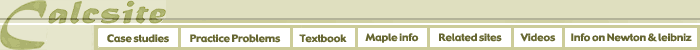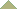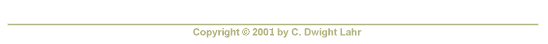The problems corresponding to each section of the book Principles of Calculus Modeling: An Interactive Approach by Donald Kreider and Dwight Lahr can be found by logging onto WeBWorK.

 Book Section WeBWorK Problems Description 1.1 Set 101 Modeling discrete data: introduction. 1.2 Set 102 Functions and their graphs. 1.3 Set 103 Defining new functions from old. 1.4 Set 104 Trigonometric functions. 1.5 Set 105 Exponential and logarithmic functions. 1.6 Set 106 Case Study: Modeling the AIDS data. 2.1 Set 201 Modeling rates of change: introduction. 2.2 Set 202 The legacy of Galileo and Newton. 2.3 Set 203 Limits of functions. 2.4 Set 204 Limits at infinity. 2.5 Set 205 Continuity. 2.6 Set 206 Tangent lines and their slopes. 2.7 Set 207 The derivative. 2.8 Set 208 Differentiation rules. 2.9 Set 209 Derivatives of trigonometric functions. 2.10 Set 210 The mean value theorem. 2.11 Set 211 Implicit differentiation. 2.12 Set 212 Derivatives of exponentials and logs. 2.13 Set 213 Newton's method. 2.14 Set 214 Linear approximations. 2.15 Set 215 Antiderivatives and initial value problems. 2.16 Set 216 Velocity and acceleration. 2.17 Set 217 Case Study: Torricelli's Law. 3.1 Set 301 Modeling with differential equations: introduction. 3.2 Set 302 Exponential growth and decay. 3.3 Set 303 Separable differential equations. 3.4 Set 304 Slope fields and Euler's method. 3.5 Set 305 Issues in curve sketching. 3.6 Set 306 Case Study: Population Modeling. 4.1 Set 401 Modeling accumulations: introduction. 4.2 Set 402 The definite integral. 4.3 Set 403 Properties of the definite integral. 4.4 Set 404 The fundamental theorem of calculus. 4.5 Set 405 Techniques of integration. 4.6 Set 406 Trapezoid rule. 4.7 Set 407 Areas between curves. 4.8 Set 408 Volumes of solids of revolution. 4.9 Set 409 Arc length. 4.10 Set 410 Inverse trigonometric functions. 4.11 Set 411 Case Study: Flood Watch. 5.01 Set 501 Sleuthing Galileo.